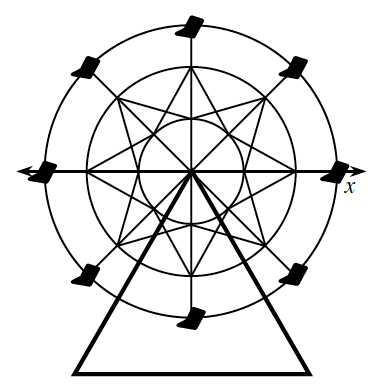### Home > CCA2 > Chapter 8 > Lesson 8.1.1 > Problem8-25

8-25.THE COUNTY FAIR FERRIS WHEEL

Consider this picture of a Ferris wheel. The wheel has a 60-foot diameter and is drawn on a set of axes with the Ferris wheel's hub (center) at the origin. Use a table like the one below and draw a graph that relates the angle (in standard position) of the spoke leading to your seat to the approximate height of the top of your seat above or below the height of the central hub. The table below starts at −90°, your starting position before you ride around the wheel.

 x (angle) y (height) $–90°$ $– 45°$ $0°$ $45°$ $90°$ $135°$ $180°$ $....$ $270°$ $–30'$
1. The wheel goes around (counter-clockwise) several times during a ride. How could you reflect this fact in your graph? Update your graph.

Include angle measures greater than $360°$ in your graph.

Draw a triangle at $-45°$ and solve for the height. The hypotenuse(radius) is $30'$.

2. What is the maximum distance above or below the center that the top of your seat attains during the ride?

$30'$

3. Find an equation to fit the County Fair Ferris wheel ride.

$y = 30 · \sin x$